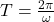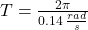## It is proposed that future space stations create an artificial gravity by rotating. Suppose a space station is constructed as a 1000-m-diame

Question

It is proposed that future space stations create an artificial gravity by rotating. Suppose a space station is constructed as a 1000-m-diameter cylinder that rotates about its axis. The inside surface is the deck of the space station. What rotation period will provide “normal” gravity?

in progress 0
2 months 2021-07-31T16:26:06+00:00 2 Answers 4 views 0

1. To solve this problem, apply the concepts related to the centripetal acceleration as the equivalent of gravity, and the kinematic equations of linear motion that will relate the speed, the distance traveled and the period of the body to meet the needs given in the problem. Centripetal acceleration is defined as,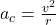Here,

v = Tangential Velocity

If we rearrange the equation to get the velocity we have,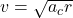But at this case the centripetal acceleration must be equal to the gravitational at the Earth, then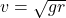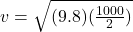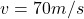The perimeter of the cylinder would be given by,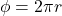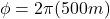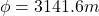Therefore now related by kinematic equations of linear motion the speed with the distance traveled and the time we will have to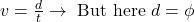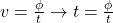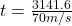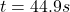Therefore the period will be 44.9s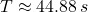Explanation:

“Normal” gravity is equal to 9.807 meters per squared second and cylinder must rotate at constant speed in order to simplify the equation of acceleration, which is in the radial direction. The centrifugal acceleration experimented by people allow them to be on the inside surface.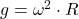The angular speed required to provide “normal” gravity is: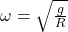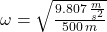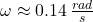The rotation period is: